xEuclidean geometryOverview

Euclidean geometry is a mathematical system attributed to the Alexandria
Alexandria
Alexandria is the second-largest city of Egypt, with a population of 4.1 million, extending about along the coast of the Mediterranean Sea in the north central part of the country; it is also the largest city lying directly on the Mediterranean coast. It is Egypt's largest seaport, serving...

n Greek mathematician
Greek mathematics
Greek mathematics, as that term is used in this article, is the mathematics written in Greek, developed from the 7th century BC to the 4th century AD around the Eastern shores of the Mediterranean. Greek mathematicians lived in cities spread over the entire Eastern Mediterranean, from Italy to...

Euclid
Euclid
Euclid , fl. 300 BC, also known as Euclid of Alexandria, was a Greek mathematician, often referred to as the "Father of Geometry". He was active in Alexandria during the reign of Ptolemy I...

, which he described in his textbook on geometry
Geometry
Geometry arose as the field of knowledge dealing with spatial relationships. Geometry was one of the two fields of pre-modern mathematics, the other being the study of numbers ....

: the Elements
Euclid's Elements
Euclid's Elements is a mathematical and geometric treatise consisting of 13 books written by the Greek mathematician Euclid in Alexandria c. 300 BC. It is a collection of definitions, postulates , propositions , and mathematical proofs of the propositions...

. Euclid's method consists in assuming a small set of intuitively appealing axiom
Axiom
In traditional logic, an axiom or postulate is a proposition that is not proven or demonstrated but considered either to be self-evident or to define and delimit the realm of analysis. In other words, an axiom is a logical statement that is assumed to be true...

s, and deducing many other proposition
Proposition
In logic and philosophy, the term proposition refers to either the "content" or "meaning" of a meaningful declarative sentence or the pattern of symbols, marks, or sounds that make up a meaningful declarative sentence...

s (theorem
Theorem
In mathematics, a theorem is a statement that has been proven on the basis of previously established statements, such as other theorems, and previously accepted statements, such as axioms...

s) from these. Although many of Euclid's results had been stated by earlier mathematicians, Euclid was the first to show how these propositions could fit into a comprehensive deductive and logical system.Unanswered QuestionsShow that euclidean space is a metric space?proof by using the properties of metric space.WHAT IS MEAN OF CONSISTENCY OF POSTULATE IN EULIDEAN GEOMETRYDiscussionsDeriving the equation of points for exact fitting and shape analysisEncyclopedia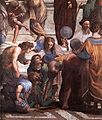Euclidean geometry is a mathematical system attributed to the Alexandria
Alexandria
Alexandria is the second-largest city of Egypt, with a population of 4.1 million, extending about along the coast of the Mediterranean Sea in the north central part of the country; it is also the largest city lying directly on the Mediterranean coast. It is Egypt's largest seaport, serving...

n Greek mathematician
Greek mathematics
Greek mathematics, as that term is used in this article, is the mathematics written in Greek, developed from the 7th century BC to the 4th century AD around the Eastern shores of the Mediterranean. Greek mathematicians lived in cities spread over the entire Eastern Mediterranean, from Italy to...

Euclid
Euclid
Euclid , fl. 300 BC, also known as Euclid of Alexandria, was a Greek mathematician, often referred to as the "Father of Geometry". He was active in Alexandria during the reign of Ptolemy I...

, which he described in his textbook on geometry
Geometry
Geometry arose as the field of knowledge dealing with spatial relationships. Geometry was one of the two fields of pre-modern mathematics, the other being the study of numbers ....

: the Elements
Euclid's Elements
Euclid's Elements is a mathematical and geometric treatise consisting of 13 books written by the Greek mathematician Euclid in Alexandria c. 300 BC. It is a collection of definitions, postulates , propositions , and mathematical proofs of the propositions...

. Euclid's method consists in assuming a small set of intuitively appealing axiom
Axiom
In traditional logic, an axiom or postulate is a proposition that is not proven or demonstrated but considered either to be self-evident or to define and delimit the realm of analysis. In other words, an axiom is a logical statement that is assumed to be true...

s, and deducing many other proposition
Proposition
In logic and philosophy, the term proposition refers to either the "content" or "meaning" of a meaningful declarative sentence or the pattern of symbols, marks, or sounds that make up a meaningful declarative sentence...

s (theorem
Theorem
In mathematics, a theorem is a statement that has been proven on the basis of previously established statements, such as other theorems, and previously accepted statements, such as axioms...

s) from these. Although many of Euclid's results had been stated by earlier mathematicians, Euclid was the first to show how these propositions could fit into a comprehensive deductive and logical system. The Elements begins with plane geometry, still taught in secondary school
Secondary school
Secondary school is a term used to describe an educational institution where the final stage of schooling, known as secondary education and usually compulsory up to a specified age, takes place...

as the first axiomatic system
Axiomatic system
In mathematics, an axiomatic system is any set of axioms from which some or all axioms can be used in conjunction to logically derive theorems. A mathematical theory consists of an axiomatic system and all its derived theorems...

and the first examples of formal proof
Mathematical proof
In mathematics, a proof is a convincing demonstration that some mathematical statement is necessarily true. Proofs are obtained from deductive reasoning, rather than from inductive or empirical arguments. That is, a proof must demonstrate that a statement is true in all cases, without a single...

. It goes on to the solid geometry
Solid geometry
In mathematics, solid geometry was the traditional name for the geometry of three-dimensional Euclidean space — for practical purposes the kind of space we live in. It was developed following the development of plane geometry...

of three dimensions. Much of the Elements states results of what are now called algebra
Algebra
Algebra is the branch of mathematics concerning the study of the rules of operations and relations, and the constructions and concepts arising from them, including terms, polynomials, equations and algebraic structures...

and number theory
Number theory
Number theory is a branch of pure mathematics devoted primarily to the study of the integers. Number theorists study prime numbers as well...

, couched in geometrical language.

For over two thousand years, the adjective "Euclidean" was unnecessary because no other sort of geometry had been conceived. Euclid's axioms seemed so intuitively obvious that any theorem proved from them was deemed true in an absolute, often metaphysical, sense. Today, however, many other self-consistent non-Euclidean geometries
Non-Euclidean geometry
Non-Euclidean geometry is the term used to refer to two specific geometries which are, loosely speaking, obtained by negating the Euclidean parallel postulate, namely hyperbolic and elliptic geometry. This is one term which, for historical reasons, has a meaning in mathematics which is much...

are known, the first ones having been discovered in the early 19th century. An implication of Einstein's theory of general relativity
General relativity
General relativity or the general theory of relativity is the geometric theory of gravitation published by Albert Einstein in 1916. It is the current description of gravitation in modern physics...

is that Euclidean space
Euclidean space
In mathematics, Euclidean space is the Euclidean plane and three-dimensional space of Euclidean geometry, as well as the generalizations of these notions to higher dimensions...

is a good approximation to the properties of physical space only where the gravitational field is not too strong.

## The Elements

The Elements are mainly a systematization of earlier knowledge of geometry. Its superiority over earlier treatments was rapidly recognized, with the result that there was little interest in preserving the earlier ones, and they are now nearly all lost.

Books I-IV and VI discuss plane geometry. Many results about plane figures are proved, e.g., If a triangle has two equal angles, then the sides subtended by the angles are equal. The Pythagorean theorem
Pythagorean theorem
In mathematics, the Pythagorean theorem or Pythagoras' theorem is a relation in Euclidean geometry among the three sides of a right triangle...

is proved.

Books V and VII-X deal with number theory, with numbers treated geometrically via their representation as line segments with various lengths. Notions such as prime numbers and rational
Rational number
In mathematics, a rational number is any number that can be expressed as the quotient or fraction a/b of two integers, with the denominator b not equal to zero. Since b may be equal to 1, every integer is a rational number...

and irrational number
Irrational number
In mathematics, an irrational number is any real number that cannot be expressed as a ratio a/b, where a and b are integers, with b non-zero, and is therefore not a rational number....

s are introduced. The infinitude of prime numbers is proved.

Books XI-XIII concern solid geometry. A typical result is the 1:3 ratio between the volume of a cone and a cylinder with the same height and base.### Axioms

Euclidean geometry is an axiomatic system
Axiomatic system
In mathematics, an axiomatic system is any set of axioms from which some or all axioms can be used in conjunction to logically derive theorems. A mathematical theory consists of an axiomatic system and all its derived theorems...

, in which all theorems ("true statements") are derived from a small number of axioms. Near the beginning of the first book of the Elements, Euclid gives five postulates (axioms) for plane geometry, stated in terms of constructions (as translated by Thomas Heath):

"Let the following be postulated":
1. "To draw a straight line from any point
Point (geometry)
In geometry, topology and related branches of mathematics a spatial point is a primitive notion upon which other concepts may be defined. In geometry, points are zero-dimensional; i.e., they do not have volume, area, length, or any other higher-dimensional analogue. In branches of mathematics...

to any point."
2. "To produce [extend] a finite straight line
Line segment
In geometry, a line segment is a part of a line that is bounded by two end points, and contains every point on the line between its end points. Examples of line segments include the sides of a triangle or square. More generally, when the end points are both vertices of a polygon, the line segment...

continuously in a straight line."
3. "To describe a circle
Circle
A circle is a simple shape of Euclidean geometry consisting of those points in a plane that are a given distance from a given point, the centre. The distance between any of the points and the centre is called the radius....

with any centre and distance [radius]."
4. "That all right angles are equal to one another."
5. The parallel postulate
Parallel postulate
In geometry, the parallel postulate, also called Euclid's fifth postulate because it is the fifth postulate in Euclid's Elements, is a distinctive axiom in Euclidean geometry...

: "That, if a straight line falling on two straight lines make the interior angles on the same side less than two right angles, the two straight lines, if produced indefinitely, meet on that side on which are the angles less than the two right angles."

Although Euclid's statement of the postulates only explicitly asserts the existence of the constructions, they are also taken to be unique.

The Elements also include the following five "common notions":
1. Things that are equal to the same thing are also equal to one another.
2. If equals are added to equals, then the wholes are equal.
3. If equals are subtracted from equals, then the remainders are equal.
4. Things that coincide with one another equal one another.
5. The whole is greater than the part.

### The parallel postulate

To the ancients, the parallel postulate seemed less obvious than the others. Euclid himself seems to have considered it as being qualitatively different from the others, as evidenced by the organization of the Elements: the first 28 propositions he presents are those that can be proved without it.

Many alternative axioms can be formulated that have the same logical consequences as the parallel postulate. For example Playfair's axiom
Playfair's axiom
Playfair's axiom is a geometrical axiom, intended to replace the fifth postulate of Euclides :Given a line and a point not on it, at most one parallel to the given line can be drawn through the point....

states:
In a plane, through a point not on a given straight line, at most one line can be drawn that never meets the given line.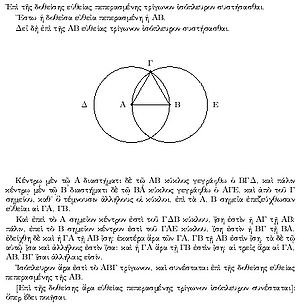## Methods of proof

Euclidean geometry is constructive
Constructive proof
In mathematics, a constructive proof is a method of proof that demonstrates the existence of a mathematical object with certain properties by creating or providing a method for creating such an object...

. Postulates 1, 2, 3, and 5 assert the existence and uniqueness of certain geometric figures, and these assertions are of a constructive nature: that is, we are not only told that certain things exist, but are also given methods for creating them with no more than a compass and an unmarked straightedge
Compass and straightedge
Compass-and-straightedge or ruler-and-compass construction is the construction of lengths, angles, and other geometric figures using only an idealized ruler and compass....

. In this sense, Euclidean geometry is more concrete than many modern axiomatic systems such as set theory
Set theory
Set theory is the branch of mathematics that studies sets, which are collections of objects. Although any type of object can be collected into a set, set theory is applied most often to objects that are relevant to mathematics...

, which often assert the existence of objects without saying how to construct them, or even assert the existence of objects that cannot be constructed within the theory. Strictly speaking, the lines on paper are models
Scientific modelling
Scientific modelling is the process of generating abstract, conceptual, graphical and/or mathematical models. Science offers a growing collection of methods, techniques and theory about all kinds of specialized scientific modelling...

of the objects defined within the formal system, rather than instances of those objects. For example a Euclidean straight line has no width, but any real drawn line will. Although nonconstructive methods
Existence theorem
In mathematics, an existence theorem is a theorem with a statement beginning 'there exist ..', or more generally 'for all x, y, ... there exist ...'. That is, in more formal terms of symbolic logic, it is a theorem with a statement involving the existential quantifier. Many such theorems will not...

are today considered by nearly all mathematicians to be just as sound as constructive ones, Euclid's constructive proofs often supplanted fallacious nonconstructive ones, e.g., some of the Pythagoreans' proofs involving irrational numbers, which usually required a statement such as "Find the greatest common measure of ..."

Euclid often used proof by contradiction. Euclidean geometry also allows the method of superposition, in which a figure is transferred to another point in space. For example, proposition I.4, side-angle-side congruence of triangles, is proved by moving one of the two triangles so that one of its sides coincides with the other triangle's equal side, and then proving that the other sides coincide as well. Some modern treatments add a sixth postulate, the rigidity of the triangle, which can be used as an alternative to superposition.

## System of measurement and arithmetic

Euclidean geometry has two fundamental types of measurements: angle and distance. The angle scale is absolute, and Euclid uses the right angle as his basic unit, so that, e.g., a 45-degree angle would be referred to as half of a right angle. The distance scale is relative; one arbitrarily picks a line segment with a certain length as the unit, and other distances are expressed in relation to it.

A line in Euclidean geometry is a model of the real number line. A line segment is a part of a line that is bounded by two end points, and contains every point on the line between its end points. Addition is represented by a construction in which one line segment is copied onto the end of another line segment to extend its length, and similarly for subtraction.

Measurements of area and volume are derived from distances. For example, a rectangle with a width of 3 and a length of 4 has an area that represents the product, 12. Because this geometrical interpretation of multiplication was limited to three dimensions, there was no direct way of interpreting the product of four or more numbers, and Euclid avoided such products, although they are implied, e.g., in the proof of book IX, proposition 20.Euclid refers to a pair of lines, or a pair of planar or solid figures, as "equal" (ἴσος) if their lengths, areas, or volumes are equal, and similarly for angles. The stronger term "congruent
Congruence (geometry)
In geometry, two figures are congruent if they have the same shape and size. This means that either object can be repositioned so as to coincide precisely with the other object...

" refers to the idea that an entire figure is the same size and shape as another figure. Alternatively, two figures are congruent if one can be moved on top of the other so that it matches up with it exactly. (Flipping it over is allowed.) Thus, for example, a 2x6 rectangle and a 3x4 rectangle are equal but not congruent, and the letter R is congruent to its mirror image. Figures that would be congruent except for their differing sizes are referred to as similar.

### Naming of points and figures

Points are customarily named using capital letters of the alphabet. Other figures, such as lines, triangles, or circles, are named by listing a sufficient number of points to pick them out unambiguously from the relevant figure, e.g., triangle ABC would typically be a triangle with vertices at points A, B, and C.

### Complementary and supplementary angles

Angles whose sum is a right angle are called complementary
Complementary angles
In geometry, complementary angles are angles whose measures sum to 90°. If the two complementary angles are adjacent their non-shared sides form a right angle....

, those whose sum is a straight angle are supplementary
Supplementary angles
Supplementary angles are pairs of angles that add up to 180 degrees. Thus the supplement of an angle of x degrees is an angle of degrees....

.

### Modern versions of Euclid's notation

In modern terminology, angles would normally be measured in degree
Degree (angle)
A degree , usually denoted by ° , is a measurement of plane angle, representing 1⁄360 of a full rotation; one degree is equivalent to π/180 radians...

s or radians.

Modern school textbooks often define separate figures called line
Line (geometry)
The notion of line or straight line was introduced by the ancient mathematicians to represent straight objects with negligible width and depth. Lines are an idealization of such objects...

s (infinite), rays (semi-infinite), and line segment
Line segment
In geometry, a line segment is a part of a line that is bounded by two end points, and contains every point on the line between its end points. Examples of line segments include the sides of a triangle or square. More generally, when the end points are both vertices of a polygon, the line segment...

s (of finite length). Euclid, rather than discussing a ray as an object that extends to infinity in one direction, would normally use locutions such as "if the line is extended to a sufficient length," although he occasionally referred to "infinite lines." A "line" in Euclid could be either straight or curved, and he used the more specific term "straight line" when necessary.

### The Bridge of Asses

The Bridge of Asses
Pons asinorum
Pons asinorum is the name given to Euclid's fifth proposition in Book 1 of his Elements of geometry, also known as the theorem on isosceles triangles. It states that the angles opposite the equal sides of an isosceles triangle are equal...

(Pons Asinorum) states that in isosceles triangles the angles at the base equal one another, and, if the equal straight lines are produced further, then the angles under the base equal one another. Its name may be attributed to its frequent role as the first real test in the Elements of the intelligence of the reader and as a bridge to the harder propositions that followed. It might also be so named because of the geometrical figure's resemblance to a steep bridge which could only be crossed by a sure–footed donkey.

### Congruence of triangles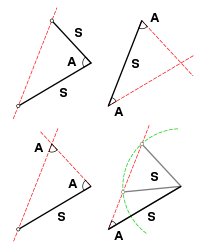Triangles are congruent if they have all three sides equal (SSS), two sides and the angle between them equal (SAS), or two angles and a side equal (ASA) (Book I, propositions 4, 8, and 26). (Triangles with three equal angles are generally similar, but not necessarily congruent. Also, triangles with two equal sides and an adjacent angle are not necessarily equal.)

### Sum of the angles of a triangle

The sum of the angles of a triangle is equal to straight angle (180 degrees).

### The Pythagorean theorem

The celebrated Pythagorean theorem
Pythagorean theorem
In mathematics, the Pythagorean theorem or Pythagoras' theorem is a relation in Euclidean geometry among the three sides of a right triangle...

(book I, proposition 47) states that in any right triangle, the area of the square whose side is the hypotenuse (the side opposite the right angle) is equal to the sum of the areas of the squares whose sides are the two legs (the two sides that meet at a right angle).

### Thales' theorem

Thales' theorem
Thales' theorem
In geometry, Thales' theorem states that if A, B and C are points on a circle where the line AC is a diameter of the circle, then the angle ABC is a right angle. Thales' theorem is a special case of the inscribed angle theorem...

, named after Thales of Miletus states that if A, B, and C are points on a circle where the line AC is a diameter of the circle, then the angle ABC is a right angle. Cantor supposed that Thales proved his theorem by means of Euclid book I, prop 32 after the manner of Euclid book III, prop 31. Tradition has it that Thales sacrificed an ox to celebrate this theorem.

### Scaling of area and volume

In modern terminology, the area of a plane figure is proportional to the square of any of its linear dimensions,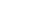, and the volume of a solid to the cube,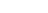. Euclid proved these results in various special cases such as the area of a circle and the volume of a parallelepipedal solid. Euclid determined some, but not all, of the relevant constants of proportionality. E.g., it was his successor Archimedes
Archimedes
Archimedes of Syracuse was a Greek mathematician, physicist, engineer, inventor, and astronomer. Although few details of his life are known, he is regarded as one of the leading scientists in classical antiquity. Among his advances in physics are the foundations of hydrostatics, statics and an...

who proved that a sphere has 2/3 the volume of the circumscribing cylinder.

## Applications

Because of Euclidean geometry's fundamental status in mathematics, it would be impossible to give more than a representative sampling of applications here.
As suggested by the etymology of the word, one of the earliest reasons for interest in geometry was surveying
Surveying
See Also: Public Land Survey SystemSurveying or land surveying is the technique, profession, and science of accurately determining the terrestrial or three-dimensional position of points and the distances and angles between them...

, and certain practical results from Euclidean geometry, such as the right-angle property of the 3-4-5 triangle, were used long before they were proved formally. The fundamental types of measurements in Euclidean geometry are distances and angles, and both of these quantities can be measured directly by a surveyor. Historically, distances were often measured by chains such as Gunter's chain
Gunter's chain
Gunter's chain is a measuring device used for land survey. It was designed and introduced in 1620 by English clergyman and mathematician Edmund Gunter long before the development of the theodolite and other more sophisticated equipment, enabling plots of land to be accurately surveyed and plotted,...

, and angles using graduated circles and, later, the theodolite
Theodolite
A theodolite is a precision instrument for measuring angles in the horizontal and vertical planes. Theodolites are mainly used for surveying applications, and have been adapted for specialized purposes in fields like metrology and rocket launch technology...

.

An application of Euclidean solid geometry is the determination of packing arrangements
Packing problem
Packing problems are a class of optimization problems in mathematics which involve attempting to pack objects together , as densely as possible. Many of these problems can be related to real life packaging, storage and transportation issues...

, such as the problem of finding the most efficient packing of spheres
Sphere packing
In geometry, a sphere packing is an arrangement of non-overlapping spheres within a containing space. The spheres considered are usually all of identical size, and the space is usually three-dimensional Euclidean space...

in n dimensions. This problem has applications in error detection and correction
Error detection and correction
In information theory and coding theory with applications in computer science and telecommunication, error detection and correction or error control are techniques that enable reliable delivery of digital data over unreliable communication channels...

.

Geometric optics uses Euclidean geometry to analyze the focusing of light by lenses and mirrors.
Geometry is used extensively in architecture
Architecture
Architecture is both the process and product of planning, designing and construction. Architectural works, in the material form of buildings, are often perceived as cultural and political symbols and as works of art...

.

Geometry can be used to design origami
Origami
is the traditional Japanese art of paper folding, which started in the 17th century AD at the latest and was popularized outside Japan in the mid-1900s. It has since then evolved into a modern art form...

. Some classical construction problems of geometry are impossible using compass and straightedge
Compass and straightedge
Compass-and-straightedge or ruler-and-compass construction is the construction of lengths, angles, and other geometric figures using only an idealized ruler and compass....

, but can be solved using origami
Mathematics of paper folding
The art of origami or paper folding has received a considerable amount of mathematical study. Fields of interest include a given paper model's flat-foldability and the use of paper folds to solve mathematical equations.-Flat folding:The construction of origami models is sometimes shown as crease...

.

## As a description of the structure of space

Euclid believed that his axioms were self-evident statements about physical reality. Euclid's proofs depend upon assumptions perhaps not obvious in Euclid's fundamental axioms, in particular that certain movements of figures do not change their geometrical properties such as the lengths of sides and interior angles, the so-called Euclidean motions, which include translations and rotations of figures.
Taken as a physical description of space, postulate 2 ( a line) asserts that space does not have holes or boundaries (in other words, space is homogeneous and unbounded); postulate 4 (equality of right angles) says that space is isotropic and figures may be moved to any location while maintaining congruence; and postulate 5 (the parallel postulate) that space is flat (has no intrinsic curvature).

As discussed in more detail below, Einstein's theory of relativity significantly modifies this view.

The ambiguous character of the axioms as originally formulated by Euclid makes it possible for different commentators to disagree about some of their other implications for the structure of space, such as whether or not it is infinite (see below) and what its topology
Topology
Topology is a major area of mathematics concerned with properties that are preserved under continuous deformations of objects, such as deformations that involve stretching, but no tearing or gluing...

is. Modern, more rigorous reformulations of the system typically aim for a cleaner separation of these issues. Interpreting Euclid's axioms in the spirit of this more modern approach, axioms 1-4 are consistent with either infinite or finite space (as in elliptic geometry
Elliptic geometry
Elliptic geometry is a non-Euclidean geometry, in which, given a line L and a point p outside L, there exists no line parallel to L passing through p. Elliptic geometry, like hyperbolic geometry, violates Euclid's parallel postulate, which can be interpreted as asserting that there is exactly one...

), and all five axioms are consistent with a variety of topologies (e.g., a plane, a cylinder, or a torus
Torus
In geometry, a torus is a surface of revolution generated by revolving a circle in three dimensional space about an axis coplanar with the circle...

for two-dimensional Euclidean geometry).

### Archimedes and Apollonius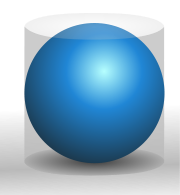Archimedes
Archimedes
Archimedes of Syracuse was a Greek mathematician, physicist, engineer, inventor, and astronomer. Although few details of his life are known, he is regarded as one of the leading scientists in classical antiquity. Among his advances in physics are the foundations of hydrostatics, statics and an...

(ca. 287 BCE – ca. 212 BCE), a colorful figure about whom many historical anecdotes are recorded, is remembered along with Euclid as one of the greatest of ancient mathematicians. Although the foundations of his work were put in place by Euclid, his work, unlike Euclid's, is believed to have been entirely original. He proved equations for the volumes and areas of various figures in two and three dimensions, and enunciated the Archimedean property
Archimedean property
In abstract algebra and analysis, the Archimedean property, named after the ancient Greek mathematician Archimedes of Syracuse, is a property held by some ordered or normed groups, fields, and other algebraic structures. Roughly speaking, it is the property of having no infinitely large or...

of finite numbers.

Apollonius of Perga
Apollonius of Perga
Apollonius of Perga [Pergaeus] was a Greek geometer and astronomer noted for his writings on conic sections. His innovative methodology and terminology, especially in the field of conics, influenced many later scholars including Ptolemy, Francesco Maurolico, Isaac Newton, and René Descartes...

(ca. 262 BCE–ca. 190 BCE) is mainly known for his investigation of conic sections.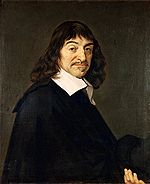### The 17th century: Descartes

René Descartes
René Descartes
René Descartes ; was a French philosopher and writer who spent most of his adult life in the Dutch Republic. He has been dubbed the 'Father of Modern Philosophy', and much subsequent Western philosophy is a response to his writings, which are studied closely to this day...

(1596–1650) developed analytic geometry
Analytic geometry
Analytic geometry, or analytical geometry has two different meanings in mathematics. The modern and advanced meaning refers to the geometry of analytic varieties...

, an alternative method for formalizing geometry. In this approach, a point is represented by its Cartesian
Cartesian coordinate system
A Cartesian coordinate system specifies each point uniquely in a plane by a pair of numerical coordinates, which are the signed distances from the point to two fixed perpendicular directed lines, measured in the same unit of length...

(x, y) coordinates, a line is represented by its equation, and so on. In Euclid's original approach, the Pythagorean theorem
Pythagorean theorem
In mathematics, the Pythagorean theorem or Pythagoras' theorem is a relation in Euclidean geometry among the three sides of a right triangle...

follows from Euclid's axioms. In the Cartesian approach, the axioms are the axioms of algebra, and the equation expressing the Pythagorean theorem is then a definition of one of the terms in Euclid's axioms, which are now considered to be theorems. The equation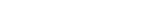defining the distance between two points P = (p, q) and Q=(r, s) is then known as the Euclidean metric
Metric space
In mathematics, a metric space is a set where a notion of distance between elements of the set is defined.The metric space which most closely corresponds to our intuitive understanding of space is the 3-dimensional Euclidean space...

, and other metrics define non-Euclidean geometries
Non-Euclidean geometry
Non-Euclidean geometry is the term used to refer to two specific geometries which are, loosely speaking, obtained by negating the Euclidean parallel postulate, namely hyperbolic and elliptic geometry. This is one term which, for historical reasons, has a meaning in mathematics which is much...

.

In terms of analytic geometry, the restriction of classical geometry to compass and straightedge constructions means a restriction to first- and second-order equations, e.g., y = 2x + 1 (a line), or x2 + y2 = 7 (a circle).

Also in the 17th century, Girard Desargues, motivated by the theory of perspective
Perspective (graphical)
Perspective in the graphic arts, such as drawing, is an approximate representation, on a flat surface , of an image as it is seen by the eye...

, introduced the concept of idealized points, lines, and planes at infinity. The result can be considered as a type of generalized geometry, projective geometry
Projective geometry
In mathematics, projective geometry is the study of geometric properties that are invariant under projective transformations. This means that, compared to elementary geometry, projective geometry has a different setting, projective space, and a selective set of basic geometric concepts...

, but it can also be used to produce proofs in ordinary Euclidean geometry in which the number of special cases is reduced.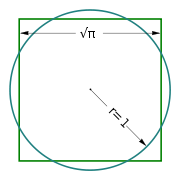### The 18th century

Geometers of the 18th century struggled to define the boundaries of the Euclidean system. Many tried in vain to prove the fifth postulate from the first four. By 1763 at least 28 different proofs had been published, but all were found to be incorrect.

Leading up to this period, geometers also tried to determine what constructions could be accomplished in Euclidean geometry. For example, the problem of trisecting an angle with a compass and straightedge is one that naturally occurs within the theory, since the axioms refer to constructive operations that can be carried out with those tools. However, centuries of efforts failed to find a solution to this problem, until Pierre Wantzel
Pierre Wantzel
Pierre Laurent Wantzel was a French mathematician who proved that several ancient geometric problems were impossible to solve using only compass and straightedge....

published a proof in 1837 that such a construction was impossible. Other constructions that were proved to be impossible include doubling the cube
Doubling the cube
Doubling the cube is one of the three most famous geometric problems unsolvable by compass and straightedge construction...

and squaring the circle
Squaring the circle
Squaring the circle is a problem proposed by ancient geometers. It is the challenge of constructing a square with the same area as a given circle by using only a finite number of steps with compass and straightedge...

. In the case of doubling the cube, the impossibility of the construction originates from the fact that the compass and straightedge method involve first- and second-order equations, while doubling a cube requires the solution of a third-order equation.

Euler
Leonhard Euler
Leonhard Euler was a pioneering Swiss mathematician and physicist. He made important discoveries in fields as diverse as infinitesimal calculus and graph theory. He also introduced much of the modern mathematical terminology and notation, particularly for mathematical analysis, such as the notion...

discussed a generalization of Euclidean geometry called affine geometry
Affine geometry
In mathematics affine geometry is the study of geometric properties which remain unchanged by affine transformations, i.e. non-singular linear transformations and translations...

, which retains the fifth postulate unmodified while weakening postulates three and four in a way that eliminates the notions of angle (whence right triangles become meaningless) and of equality of length of line segments in general (whence circles become meaningless) while retaining the notions of parallelism as an equivalence relation between lines, and equality of length of parallel line segments (so line segments continue to have a midpoint).

### The 19th century and non-Euclidean geometry

In the early 19th century, Carnot
Lazare Carnot
Lazare Nicolas Marguerite, Comte Carnot , the Organizer of Victory in the French Revolutionary Wars, was a French politician, engineer, and mathematician.-Education and early life:...

and Möbius
August Ferdinand Möbius
August Ferdinand Möbius was a German mathematician and theoretical astronomer.He is best known for his discovery of the Möbius strip, a non-orientable two-dimensional surface with only one side when embedded in three-dimensional Euclidean space. It was independently discovered by Johann Benedict...

systematically developed the use of signed angles and line segments as a way of simplifying and unifying results.

The century's most significant development in geometry occurred when, around 1830, János Bolyai
János Bolyai
János Bolyai was a Hungarian mathematician, known for his work in non-Euclidean geometry.Bolyai was born in the Transylvanian town of Kolozsvár , then part of the Habsburg Empire , the son of Zsuzsanna Benkő and the well-known mathematician Farkas Bolyai.-Life:By the age of 13, he had mastered...

and Nikolai Ivanovich Lobachevsky
Nikolai Ivanovich Lobachevsky
Nikolai Ivanovich Lobachevsky was a Russian mathematician and geometer, renowned primarily for his pioneering works on hyperbolic geometry, otherwise known as Lobachevskian geometry...

separately published work on non-Euclidean geometry
Non-Euclidean geometry
Non-Euclidean geometry is the term used to refer to two specific geometries which are, loosely speaking, obtained by negating the Euclidean parallel postulate, namely hyperbolic and elliptic geometry. This is one term which, for historical reasons, has a meaning in mathematics which is much...

, in which the parallel postulate is not valid. Since non-Euclidean geometry is provably self-consistent, the parallel postulate cannot be proved from the other postulates.

In the 19th century, it was also realized that Euclid's ten axioms and common notions do not suffice to prove all of theorems stated in the Elements. For example, Euclid assumed implicitly that any line contains at least two points, but this assumption cannot be proved from the other axioms, and therefore needs to be an axiom itself. The very first geometric proof in the Elements, shown in the figure above, is that any line segment is part of a triangle; Euclid constructs this in the usual way, by drawing circles around both endpoints and taking their intersection as the third vertex. His axioms, however, do not guarantee that the circles actually intersect, because they do not assert the geometrical property of continuity, which in Cartesian terms is equivalent to the completeness property of the real numbers. Starting with Moritz Pasch
Moritz Pasch
Moritz Pasch was a German mathematician specializing in the foundations of geometry. He completed his Ph.D. at the University of Breslau at only 22 years of age...

in 1882, many improved axiomatic systems for geometry have been proposed, the best known being those of Hilbert
Hilbert's axioms
Hilbert's axioms are a set of 20 assumptions proposed by David Hilbert in 1899 in his book Grundlagen der Geometrie , as the foundation for a modern treatment of Euclidean geometry...

, George Birkhoff
Birkhoff's axioms
In 1932, G. D. Birkhoff created a set of four postulates of Euclidean geometry sometimes referred to as Birkhoff's axioms. These postulates are all based on basic geometry that can be confirmed experimentally with a scale and protractor. Since the postulates build upon the real numbers, the...

, and Tarski
Tarski's axioms
Tarski's axioms, due to Alfred Tarski, are an axiom set for the substantial fragment of Euclidean geometry, called "elementary," that is formulable in first-order logic with identity, and requiring no set theory . Other modern axiomizations of Euclidean geometry are those by Hilbert and George...

.

### The 20th century and general relativity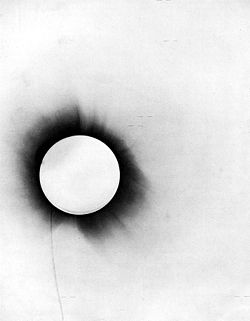Einstein's
Albert Einstein
Albert Einstein was a German-born theoretical physicist who developed the theory of general relativity, effecting a revolution in physics. For this achievement, Einstein is often regarded as the father of modern physics and one of the most prolific intellects in human history...

theory of general relativity
General relativity
General relativity or the general theory of relativity is the geometric theory of gravitation published by Albert Einstein in 1916. It is the current description of gravitation in modern physics...

shows that the true geometry of spacetime is not Euclidean geometry. For example, if a triangle is constructed out of three rays of light, then in general the interior angles do not add up to 180 degrees due to gravity. A relatively weak gravitational field, such as the Earth's or the sun's, is represented by a metric that is approximately, but not exactly, Euclidean. Until the 20th century, there was no technology capable of detecting the deviations from Euclidean geometry, but Einstein predicted that such deviations would exist. They were later verified by observations such as the slight bending of starlight by the Sun during a solar eclipse in 1919, and such considerations are now an integral part of the software that runs the GPS
Global Positioning System
The Global Positioning System is a space-based global navigation satellite system that provides location and time information in all weather, anywhere on or near the Earth, where there is an unobstructed line of sight to four or more GPS satellites...

system. It is possible to object to this interpretation of general relativity on the grounds that light rays might be improper physical models of Euclid's lines, or that relativity could be rephrased so as to avoid the geometrical interpretations. However, one of the consequences of Einstein's theory is that there is no possible physical test that can distinguish between a beam of light as a model of a geometrical line and any other physical model. The entire notion of physical tests of the axioms of geometry should be rejected, and geometry should be considered to be a formal system without any intrinsic real-world meaning.

### Infinite objects

Euclid sometimes distinguished explicitly between "finite lines" (e.g., Postulate 2) and "infinite
Infinity
Infinity is a concept in many fields, most predominantly mathematics and physics, that refers to a quantity without bound or end. People have developed various ideas throughout history about the nature of infinity...

lines" (book I, proposition 12). However, he typically did not make such distinctions unless they were necessary. The postulates do not explicitly refer to infinite lines, although for example some commentators interpret postulate 3, existence of a circle with any radius, as implying that space is infinite.

The notion of infinitesimally small quantities had previously been discussed extensively by the Eleatic School, but nobody had been able to put them on a firm logical basis, with paradoxes such as Zeno's paradox occurring that had not been resolved to universal satisfaction. Euclid used the method of exhaustion
Method of exhaustion
The method of exhaustion is a method of finding the area of a shape by inscribing inside it a sequence of polygons whose areas converge to the area of the containing shape. If the sequence is correctly constructed, the difference in area between the n-th polygon and the containing shape will...

rather than infinitesimals.

Later ancient commentators such as Proclus
Proclus
Proclus Lycaeus , called "The Successor" or "Diadochos" , was a Greek Neoplatonist philosopher, one of the last major Classical philosophers . He set forth one of the most elaborate and fully developed systems of Neoplatonism...

(410-485 CE) treated many questions about infinity as issues demanding proof and, e.g., Proclus claimed to prove the infinite divisibility of a line, based on a proof by contradiction in which he considered the cases of even and odd numbers of points constituting it.

At the turn of the 20th century, Giuseppe Veronese
Giuseppe Veronese
Giuseppe Veronese was an Italian mathematician. He was born in Chioggia, near Venice.Although his work was severely criticised as unsound by Peano, he is now recognised as having priority on many ideas that have since become parts of transfinite numbers and model theory, and as one of the...

produced controversial work on non-Archimedean
Archimedean property
In abstract algebra and analysis, the Archimedean property, named after the ancient Greek mathematician Archimedes of Syracuse, is a property held by some ordered or normed groups, fields, and other algebraic structures. Roughly speaking, it is the property of having no infinitely large or...

models of Euclidean geometry, in which the distance between two points may be infinite or infinitesimal, in the Newton
Isaac Newton
Sir Isaac Newton PRS was an English physicist, mathematician, astronomer, natural philosopher, alchemist, and theologian, who has been "considered by many to be the greatest and most influential scientist who ever lived."...

Leibniz
Gottfried Leibniz
Gottfried Wilhelm Leibniz was a German philosopher and mathematician. He wrote in different languages, primarily in Latin , French and German ....

sense. Fifty years later, Abraham Robinson
Abraham Robinson
Abraham Robinson was a mathematician who is most widely known for development of non-standard analysis, a mathematically rigorous system whereby infinitesimal and infinite numbers were incorporated into mathematics....

provided a rigorous logical foundation for Veronese's work.

### Infinite processes

One reason that the ancients treated the parallel postulate as less certain than the others is that verifying it physically would require us to inspect two lines to check that they never intersected, even at some very distant point, and this inspection could potentially take an infinite amount of time.

The modern formulation of proof by induction was not developed until the 17th century, but some later commentators consider it to be implicit in some of Euclid's proofs, e.g., the proof of the infinitude of primes.

Supposed paradoxes involving infinite series, such as Zeno's paradox, predated Euclid. Euclid avoided such discussions, giving, for example, the expression for the partial sums of the geometric series in IX.35 without commenting on the possibility of letting the number of terms become infinite.

## Logical basis

### Classical logic

Euclid frequently used the method of proof by contradiction, and therefore the traditional presentation of Euclidean geometry assumes classical logic
Classical logic
Classical logic identifies a class of formal logics that have been most intensively studied and most widely used. The class is sometimes called standard logic as well...

, in which every proposition is either true or false, i.e., for any proposition P, the proposition "P or not P" is automatically true.

### Modern standards of rigor

Placing Euclidean geometry on a solid axiomatic basis was a preoccupation of mathematicians for centuries. The role of primitive notion
Primitive notion
In mathematics, logic, and formal systems, a primitive notion is an undefined concept. In particular, a primitive notion is not defined in terms of previously defined concepts, but is only motivated informally, usually by an appeal to intuition and everyday experience. In an axiomatic theory or...

s, or undefined concepts, was clearly put forward by Alessandro Padoa
Alessandro Padoa
Alessandro Padoa was an Italian mathematician and logician, a contributor to the school of Giuseppe Peano. He is remembered for a method for deciding whether, given some formal theory, a new primitive notion is truly independent of the other primitive notions...

of the Peano
Giuseppe Peano
Giuseppe Peano was an Italian mathematician, whose work was of philosophical value. The author of over 200 books and papers, he was a founder of mathematical logic and set theory, to which he contributed much notation. The standard axiomatization of the natural numbers is named the Peano axioms in...

delegation at the 1900 Paris conference:
That is, mathematics is context-independent knowledge within a hierarchical framework. As said by Bertrand Russell:
Such foundational approaches range between foundationalism
Foundationalism
Foundationalism is any theory in epistemology that holds that beliefs are justified based on what are called basic beliefs . This position is intended to resolve the infinite regress problem in epistemology...

and formalism
Formalism (mathematics)
In foundations of mathematics, philosophy of mathematics, and philosophy of logic, formalism is a theory that holds that statements of mathematics and logic can be thought of as statements about the consequences of certain string manipulation rules....

.

### Axiomatic formulations

• Euclid's axioms: In his dissertation to Trinity College, Cambridge, Bertrand Russell summarized the changing role of Euclid's geometry in the minds of philosophers up to that time. It was a conflict between certain knowledge, independent of experiment, and empiricism, requiring experimental input. This issue became clear as it was discovered that the parallel postulate
Parallel postulate
In geometry, the parallel postulate, also called Euclid's fifth postulate because it is the fifth postulate in Euclid's Elements, is a distinctive axiom in Euclidean geometry...

was not necessarily valid and its applicability was an empirical matter, deciding whether the applicable geometry was Euclidean or non-Euclidean
Non-Euclidean geometry
Non-Euclidean geometry is the term used to refer to two specific geometries which are, loosely speaking, obtained by negating the Euclidean parallel postulate, namely hyperbolic and elliptic geometry. This is one term which, for historical reasons, has a meaning in mathematics which is much...

.
• Hilbert's axioms
Hilbert's axioms
Hilbert's axioms are a set of 20 assumptions proposed by David Hilbert in 1899 in his book Grundlagen der Geometrie , as the foundation for a modern treatment of Euclidean geometry...

: Hilbert's axioms had the goal of identifying a simple and complete set of independent axioms from which the most important geometric theorems could be deduced. The outstanding objectives were to make Euclidean geometry rigorous (avoiding hidden assumptions) and to make clear the ramifications of the parallel postulate.
• Birkhoff's axioms
Birkhoff's axioms
In 1932, G. D. Birkhoff created a set of four postulates of Euclidean geometry sometimes referred to as Birkhoff's axioms. These postulates are all based on basic geometry that can be confirmed experimentally with a scale and protractor. Since the postulates build upon the real numbers, the...

: Birkhoff proposed four postulates for Euclidean geometry that can be confirmed experimentally with scale and protractor. The notions of angle and distance become primitive concepts.
• Tarski's axioms
Tarski's axioms
Tarski's axioms, due to Alfred Tarski, are an axiom set for the substantial fragment of Euclidean geometry, called "elementary," that is formulable in first-order logic with identity, and requiring no set theory . Other modern axiomizations of Euclidean geometry are those by Hilbert and George...

:Tarski (1902–1983) and his students defined elementary Euclidean geometry as the geometry that can be expressed in first-order logic
First-order logic
First-order logic is a formal logical system used in mathematics, philosophy, linguistics, and computer science. It goes by many names, including: first-order predicate calculus, the lower predicate calculus, quantification theory, and predicate logic...

and does not depend on set theory
Set theory
Set theory is the branch of mathematics that studies sets, which are collections of objects. Although any type of object can be collected into a set, set theory is applied most often to objects that are relevant to mathematics...

for its logical basis, in contrast to Hilbert's axioms which involve point sets. Tarski proved his axiomatic formulation of elementary Euclidean geometry to be consistent and complete in a certain sense
Decidability (logic)
In logic, the term decidable refers to the decision problem, the question of the existence of an effective method for determining membership in a set of formulas. Logical systems such as propositional logic are decidable if membership in their set of logically valid formulas can be effectively...

: there is an algorithm which, for every proposition, can show it to be either true or false. (This doesn't violate Gödel's theorem
Gödel's incompleteness theorems
Gödel's incompleteness theorems are two theorems of mathematical logic that establish inherent limitations of all but the most trivial axiomatic systems capable of doing arithmetic. The theorems, proven by Kurt Gödel in 1931, are important both in mathematical logic and in the philosophy of...

, because Euclidean geometry cannot describe a sufficient amount of arithmetic for the theorem to apply.) This is equivalent to the decidability of real closed fields, of which elementary Euclidean geometry is a model.

### Constructive approaches and pedagogy

The process of abstract axiomatization as exemplified by Hilbert's axioms
Hilbert's axioms
Hilbert's axioms are a set of 20 assumptions proposed by David Hilbert in 1899 in his book Grundlagen der Geometrie , as the foundation for a modern treatment of Euclidean geometry...

reduces geometry to theorem proving or predicate logic
Predicate logic
In mathematical logic, predicate logic is the generic term for symbolic formal systems like first-order logic, second-order logic, many-sorted logic or infinitary logic. This formal system is distinguished from other systems in that its formulae contain variables which can be quantified...

. In contrast, the Greeks used construction postulates, and emphasized problem solving. For the Greeks, constructions are more primitive than existence propositions, and can be used to prove existence propositions, but not vice versa. To describe problem solving adequately requires a richer system of logical concepts. The contrast in approach may be summarized:
• Axiomatic proof: Proofs are deductive derivations of propositions from primitive premises that are ‘true’ in some sense. The aim is to justify the proposition.
• Analytic proof: Proofs are non-deductive derivations of hypothesis from problems. The aim is to find hypotheses capable of giving a solution to the problem. One can argue that Euclid's axioms were arrived upon in this manner. In particular, it is thought that Euclid felt the parallel postulate
Parallel postulate
In geometry, the parallel postulate, also called Euclid's fifth postulate because it is the fifth postulate in Euclid's Elements, is a distinctive axiom in Euclidean geometry...

was forced upon him, as indicated by his reluctance to make use of it, and his arrival upon it by the method of contradiction.

Andrei Nicholaevich Kolmogorov proposed a problem solving basis for geometry. This work was a precursor of a modern formulation in terms of constructive type theory. This development has implications for pedagogy as well.

## See also

• Analytic geometry
Analytic geometry
Analytic geometry, or analytical geometry has two different meanings in mathematics. The modern and advanced meaning refers to the geometry of analytic varieties...

• Type theory
Type theory
In mathematics, logic and computer science, type theory is any of several formal systems that can serve as alternatives to naive set theory, or the study of such formalisms in general...

• Interactive geometry software
Interactive geometry software
Interactive geometry software are computer programs which allow one to create and then manipulate geometric constructions, primarily in plane geometry. In most IGS, one starts construction by putting a few points and using them to define new objects such as lines, circles or other points...

• Non-Euclidean geometry
Non-Euclidean geometry
Non-Euclidean geometry is the term used to refer to two specific geometries which are, loosely speaking, obtained by negating the Euclidean parallel postulate, namely hyperbolic and elliptic geometry. This is one term which, for historical reasons, has a meaning in mathematics which is much...

• Ordered geometry
Ordered geometry
Ordered geometry is a form of geometry featuring the concept of intermediacy but, like projective geometry, omitting the basic notion of measurement...

• Incidence geometry
• Metric geometry
• Birkhoff's axioms
Birkhoff's axioms
In 1932, G. D. Birkhoff created a set of four postulates of Euclidean geometry sometimes referred to as Birkhoff's axioms. These postulates are all based on basic geometry that can be confirmed experimentally with a scale and protractor. Since the postulates build upon the real numbers, the...

• Hilbert's axioms
Hilbert's axioms
Hilbert's axioms are a set of 20 assumptions proposed by David Hilbert in 1899 in his book Grundlagen der Geometrie , as the foundation for a modern treatment of Euclidean geometry...

• Parallel postulate
Parallel postulate
In geometry, the parallel postulate, also called Euclid's fifth postulate because it is the fifth postulate in Euclid's Elements, is a distinctive axiom in Euclidean geometry...

• Schopenhauer's criticism of the proofs of the Parallel Postulate
Schopenhauer's criticism of the proofs of the parallel postulate
Arthur Schopenhauer criticized mathematicians' attempts to prove Euclid's Parallel Postulate because they try to prove from indirect concepts that which is directly evident from perception....

• Cartesian coordinate system
Cartesian coordinate system
A Cartesian coordinate system specifies each point uniquely in a plane by a pair of numerical coordinates, which are the signed distances from the point to two fixed perpendicular directed lines, measured in the same unit of length...

### Classical theorems

• Ceva's theorem
Ceva's theorem
Ceva's theorem is a theorem about triangles in plane geometry. Given a triangle ABC, let the lines AO, BO and CO be drawn from the vertices to a common point O to meet opposite sides at D, E and F respectively...

• Heron's formula
• Nine-point circle
Nine-point circle
In geometry, the nine-point circle is a circle that can be constructed for any given triangle. It is so named because it passes through nine significant points defined from the triangle...

• Pythagorean theorem
Pythagorean theorem
In mathematics, the Pythagorean theorem or Pythagoras' theorem is a relation in Euclidean geometry among the three sides of a right triangle...

• Menelaus' theorem
Menelaus' theorem
Menelaus' theorem, named for Menelaus of Alexandria, is a theorem about triangles in plane geometry. Given a triangle ABC, and a transversal line that crosses BC, AC and AB at points D, E and F respectively, with D, E, and F distinct from A, B and C, thenThis equation uses signed lengths of...

• Angle bisector theorem
Angle bisector theorem
In geometry, the angle bisector theorem is concerned with the relative lengths of the two segments that a triangle's side is divided into by a line that bisects the opposite angle. It equates their relative lengths to the relative lengths of the other two sides of the triangle.Consider a triangle...

• Butterfly theorem
Butterfly theorem
The butterfly theorem is a classical result in Euclidean geometry, which can be stated as follows:Let M be the midpoint of a chord PQ of a circle, through which two other chords AB and CD are drawn; AD and BC intersect chord PQ at X and Y correspondingly...

## External links

The source of this article is wikipedia, the free encyclopedia.  The text of this article is licensed under the GFDL.